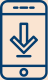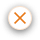#### Helping you invest with confidence# Demystifying Duration and Modified Duration for Debt Mutual Funds

22 Feb 2022 0 COMMENTWe have all heard about debt mutual funds and their benefits as an investment option. But ever wondered what are the measures that go into selecting a good debt mutual fund? Let’s explore one such interesting measure in this article and see how this term called ‘duration’ helps us determine the price change of a fixed income security.

## To begin with, let’s understand what the word ‘duration’ means

Let’s take a simple example to understand this.

Let’s assume you buy a bond of face value of 100 with a 10% coupon, which is essentially the interest rate paid by the issuer of the bond to you, with a maturity of 10 years. Now let’s say that the interest rates decline that year by 1% making it 9%, the bond yields also go down. What this means is that current issues of new bonds will offer lesser returns. Since your 10-year bond is going to pay you a higher return than a bond at today’s interest rate, investors will be willing to pay more than 100 for your 10-year 10% coupon bond. This means you can sell your bond at a higher price than what you bought it at.

Well, much in the same way, the opposite is also true. When the interest rates go up, the price effect on the bond will be the opposite.

To understand simply, there are broadly two terms here to keep in mind, bond prices and interest rates and that they share an inverse relationship. Which means that when the interest rates rise, the bond prices go down and likewise, when the interest rates drop, the bond prices go up.

But, do all the bonds show the same level of price change when the interest rate changes?

The answer is No. The price change in a bond depends on the bond’s ‘duration.' So, what is duration? It would help if you did not confuse the word duration with time to maturity. Both are two different terms but are related.

## Alright so how is duration different from maturity?

Maturity is quite straightforward. It is the amount of time in years until the principal amount is paid back. A 10-year bond like we spoke about earlier, will earn interest for 10 years and after which the principal amount is paid back to the investor of the bond.

This time period after which the principal amount is paid back to the investor is called as the maturity.

On the other hand, duration is the time in which a bond can pay back its price from its future cash flow. However, duration is directly proportional to the bond's maturity period. The longer the maturity period, the longer would be the duration of the bond.

## Now, why is this ‘duration’ important and what is its use?

Understanding duration is critical for investors that invest in debt mutual fund schemes. Simply put, duration is a measure of the interest rate risk of debt security. Much like how other investment instruments have their measures to know if they are risky or not, duration tells investors a numerically quantifiable measure for the risk a bond price entails due to change in interest rates.

If a mutual fund scheme has a lower duration, the fund’s NAV will not fluctuate much with a change in interest rate. Therefore, these funds are suitable for those investors who have a low-risk appetite.

On the other hand, if the fund’s average duration is high, it carries a high-interest risk and is preferred by risk-taking investors. As a result, these funds are comparatively more beneficial in a falling interest rate scenario. Conversely, these funds could be risky in a rising interest rate scenario.

You can choose the debt fund based on these factors, considering your risk capacity and financial goals.

## Now let’s move to something called as modified duration

Modified duration is the direct indication of a percentage change in bond price for a unit change in the interest rates.

In other words, we can say that modified duration measures the price sensitivity of a bond. For example, if a bond has a modified duration of 4%, the bond price will rise by 4% with a decrease in interest rate by 1%.  Likewise, bond prices will drop by 4% on increasing the interest rate by 1%.

In conclusion, duration and modified duration is a measure that helps investors to get an idea of how risky a fund is and helps us understand the price sensitivity of a fund based on a change in interest rates.